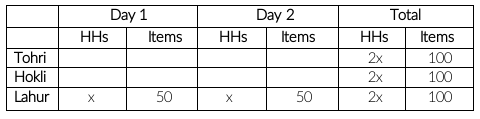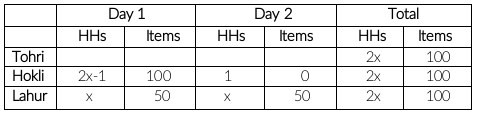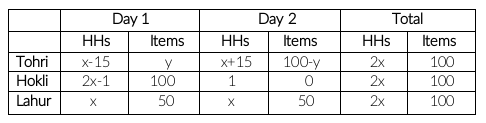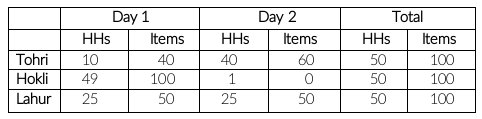### CAT 2022 Question Paper (Slot 2) Question 25

Instructions

A few salesmen are employed to sell a product called TRICCEK among households in various housing complexes. On each day, a salesman is assigned to visit one housing complex. Once a salesman enters a housing complex, he can meet any number of households in the time available. However, if a household makes a complaint against the salesman, then he must leave the housing complex immediately and cannot meet any other household on that day. A household may buy any number of TRICCEK items or may not buy any item. The salesman needs to record the total number of TRICCEK items sold as well as the number of households met in each day. The success rate of a salesman for a day is defined as the ratio of the number of items sold to the number of households met on that day. Some details about the performances of three salesmen - Tohri, Hokli and Lahur, on two particular days are given below.

1. Over the two days, all three of them met the same total number of households, and each of them sold a total of 100 items.
2. On both days, Lahur met the same number of households and sold the same number of items.
3. Hokli could not sell any item on the second day because the first household he met on that day complained against him.
4. Tohri met 30 more households on the second day than on the first day.

5. Tohri’s success rate was twice that of Lahur’s on the first day, and it was 75% of Lahur’s on the second day.

Question 25

# What was the total number of households met by Tohri, Hokli and Lahur on the first day?

Solution

In statement 1, it is given that all three of them met the same total number of households, and each of them sold a total of 100 items in two days. In statement 2, it is given that on both days, Lahur met the same number of households and sold the same number of items. This implies he sold 50 items per day. Let the number households Lahur met in a day be 'x'.
Total number of households each of them met in two days will be '2x'.In statement 3, it is given that Hokli could not sell any item on the second day because the first household he met on that day complained against him. This implies he met only 1 household on day 2.In statement 4, it is given that Tohri met 30 more households on the second day than on the first day.
Let the number of households Tohri met on day 1 be 'a'
It is given,
a + a + 30 = 2x
a + 15 = x
a = x - 15In statement 5, it is given that

$$2\left(\frac{50}{x}\right)=\frac{y}{x-15}$$ ...... (1)

$$\frac{3}{4}\left(\frac{50}{x}\right)=\ \frac{\ 100-y}{x+15}$$ ...... (2)

$$\frac{100}{x}=\ \frac{\ y}{x-15}$$

$$\frac{100}{y}=\ \frac{\ x}{x-15}$$

$$\frac{y}{100}=\ \ 1-\frac{15}{x}$$

$$x=\frac{1500}{100-y}$$

Substituting x in (2), we get

y = 40 and x = 25

Final Table:The total number of households met by Tohri, Hokli and Lahur on the first day is 10 + 49 + 25, i.e. 84.